# （一）ZOJ 1096：Subway

题意：给出下列参数（所有参数均为小于等于1000的正整数），求地铁列车从一个站点到下一个站点之间的最短时间（精确到 0.1 秒）。

d - 地铁站之间的距离（米）;

m - 列车的最大速度（米 / 秒）;

a1 - 最大加速度（绝对值）（米 / 秒^2);

j1 - 最大 jerk （绝对值）（米 / 秒^3).

备注：最后一个条件中的 Jerk 就是加速度对时间的导数：d/dt (a); 相当于列车所受的合力对时间的变化率，该限制条件用于防止车厢内站立的乘客摔倒。

分析：此题的重点在于考察数学和物理基础，编程本身倒不重要。对这道题目，要求求列车运行的最短时间，显然，列车应该尽可能快的加速到最大速度，然后尽可能快的减速到停止。这个结论是很显然的。由于这是OnlineJudge 题目，所以我们也不必此结论做严格的数学证明。（如果不是这样，即列车没有尽可能快的加速，那么其 v- t 曲线就会位于最佳曲线的下方，为了保证面积相等，则其时间一定要超过最佳曲线）

可以绘制出列车运行的速度曲线 v = v( t )，根据物理知识，上诉条件相当于下列表达式：

∫ v dt = d; (列车走过的距离，即 v( t ) 曲线和横轴围成的面积)

v ≤ m;

| d(v)/dt | ≤ a1;

| d^2(v)/dt^2 | ≤ j1;

根据前面的假设，v(t) 在起始阶段一定是以最大的 j1 在加速。因此：

（1）全力加速： v(t) = 1/2 * j1 * t^2;  曲线 v 为一段抛物线。设持续时间为 t1；加速度 a = j1 * t;

（2）当到达t1时，假设此时达到了最大加速度 a1。然后保持加速度为 a1 不变，进入匀加速直线运动阶段，此时曲线 v 为斜率为 a1 的直线。加速度 a = a1；设持续时间为 t3

（3）在此速度上继续加速，但尽全力降低加速速度，直到最大速度 m。曲线 v 依然是抛物线，只是开口方向相反。持续时间依然为 t1；加速度 a = - j1 * ( t - t1 - t3);

（4）已最大速度 m 进入匀速直线运动。曲线 v 是水平线段，加速度 a = 0；设该段的持续时间为 t2

（5）全力减速，抛物线，和（3）对称，持续时间为 t1。

（6）以最大减速速度 -a1 进行匀减速直线运动，斜率为 -a1 的直线线段，和（2）对称，持续时间为 t3。

（7）继续全力减速，直到停止在下一个站点，和（1）对称，持续时间为 t1。

注意上诉过程包含了 4 个含有参数 j1 的抛物线片段，先解释下 j1 的物理含义，假设列车运行在光滑轨道上，只受到一个牵引力，则在这些时间段上，牵引力变化的最快，加速度变化的最快（乘客最容易因为惯性发生摇晃）。物理量 Jerk 表示的是加速度变化的速度，根据牛顿第二定律，也就是物体所受合力的变化速度。例如，列车如果正在用最大牵引力进行全力加速，这时候乘客倾向于向后倾倒，乘客需要用很大力气紧紧抓住车厢内的扶手，为了让乘客保持稳定，这个牵引力不应该猛然撤去，而应该逐渐减小（相当于上面的曲线片段 3），如果在全力加速时一瞬间撤去牵引力，乘客就会感觉很不稳定很不舒服（现实生活中这种现象很常见，在列车加速时乘客会感觉非常不稳，也就是列车受力的变化速度太快，即 j 太大了，很显然实际生活中的地铁是达不到题目中要求的这么理想化的）。同理，如果列车在静止状态或者匀速状态，牵引力也应该逐渐加大，而不是一瞬间加到最大（这会对列车产生“猛推”效果，造成乘客在车厢内摇晃）。

最大加速度 a1 的物理含义表征的是牵引或者制动时的最大合力，不考虑刹车的话也就相当于发动机的最大输出牵引力（注：不是功率）。

上诉过程的速度 v（t）曲线如下图所示（注意：图形中的背景色表示的仅是片段的物理运动性质，但不代表相互衔接的曲线一定来自相同函数，下面的 case 图与此相同）：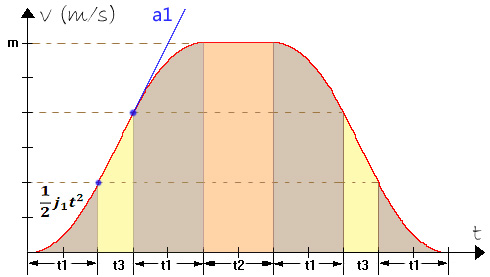很显然，曲线是关于垂直中心线轴对称的。求上诉曲线的积分即和 t 轴围成的面积很容易，抛物线由四个片段组成，它们能拼合成两个面积为 （m * t1）的矩形。斜率为 ±a1 的匀加速直线运动部分，是两个梯形，它们也能拼合成一个面积为 （m * t3）的矩形。最后中间的匀速直线运动部分，是一个面积为（m * t2）的矩形。因此，对于上诉过程有：

d = ∫ v dt = m * ( t1 * 2 + t3 + t2 );  // 两个站点之间的距离

min ( t ) = t1 * 4 + t3 * 2 + t2;          // 最短运行时间

注意：这是一种运动种类最多的“理想状况”，即距离 d 足够长，使得可以加速到最大速度 m； a1 要足够小，使得可以“割断”抛物线，出现斜线部分。实际上由于四个参数的值是任意给出的整数，这些参数可能只有一部分真正的对结果产生了限制作用，因此我们必须考虑下面的四种实际 case，如下图所示：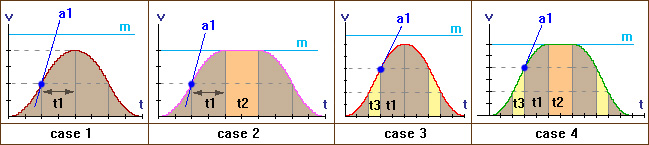（1）case 1：受到距离 d 的限制，不受 m，a1 的限制。曲线只有四个抛物线片段。

根据：∫ v dt = max(v) * t1 * 2 = (j1 * t1^2) * t1 * 2 =  d; （受到距离 d 的限制）

结果：min(t) = t1 * 4 =  [ (d / 2 j1) ^ (1/3) ] * 4;

条件1：max(v) = j1 * t1^2 ≤ m; （不会受到最大速度 m 的限制）

条件2：max(a) = j1 * t1 ≤ a1; （不会受到最大加速度 a1 的限制）

（2）case 2：受到最大速度 m 的限制，即能加速到最大速度m（此种情况可认为不受距离 d 的限制，当然也可以说 m 太小），同时也不受 a1 的限制。曲线由四个抛物线片段加一段水平线段组成。

根据：1/2 * j * (t1 ^ 2) = m / 2; （受到最大速度 m 的限制）

求出：t1 = sqrt (m / j1); 以及 t2 = (d - m * t1 * 2)/m;

结果：min(t) = t1 * 4 + t2;

条件1：m * t1 * 2 ≤ d; （不会受到距离 d 的限制）

条件2：max(a) = j1 * t1 ≤ a1; （不会受到最大加速度 a1 的限制）

（3）case 3：受到最大加速度 a1 的限制，同时受到距离 d 的限制，所以不能加速到最大速度 m （即不会受到最大速度 m 的限制）。曲线由四个抛物线片段加上两段斜率绝对值为 a1 的倾斜线段组成。则由下列方程：

j1 * t1 = a1; （受到 a1 的限制）

max(v) = j1 * (t1^2) + a * t3;

d = max(v) * (t1 * 2 + t3);

结果：min(t) = t1 * 4 + t3 * 2;

条件1：max(v) ≤ m; （不受到最大速度 m 的限制）

条件2：t3 ≥ 0; （关于 t3 的负数解在后面讨论）

整理上面的方程式，我们得到一个关于 t3 的一元二次方程如下：

a1 t3^2  + [ 3 (a1^2) / j1 ]  t3 + [ 2 ( a1^3 ) / ( j1^2 ) - d ] = 0;

可以根据一元二次方程的求根公式求出上面的 t3，解有两个，显然应该舍弃其中为负数的根，取正数根。

【注意】这里 t3 的被舍弃的负数解的数学和物理意义是什么？ 在给出代码后将继续讨论这个问题。

（4）case 4：就是前面分析过的所有运动都包括。受到 a1 的限制因此有斜线段部分，受到 m 的限制因此有水平线段部分。但不受到距离 d 的限制（即能加速到最大速度）。有：

j1 * t1 = a1; （受到最大加速度 a1 的限制）

m = j1 * (t1^2) + a * t3; （受到最大速度 m 的限制）

t2 = ( d - m * t1 * 2 - m * t3 ) / m; （不受到距离 d 的限制）

结果：min(t) = t1 * 4 + t3 * 2 + t2;

case 4 需要满足的条件实际上不需要列出，因为如果前面的case都不满足条件，则一定会落入case 4。这里为了完备，我依然给出case 4需要满足的条件：

条件1：max ( v ) / 2 = 1/2 * j1 * (t1^2) ≤ m/2; （为了能达到最大加速度a1，要有足够的加速空间，因此 m 必须足够大）。

条件2：m * ( t1*2 + t3 ) ≤ d; （为了达到最大速度，必须有足够长的距离，因此 d 必须足够大）。

/***************************************************************/

好了，现在我们已经考虑了四种 case，有没有任何遗漏的 case （要注意如果有就意味着 WA！）？考虑下斜线段出现的条件是不是一定要达到最大加速度 a1？是的，因为如果没有达到最大加速度 a1 就进入某个斜率小于 a1 的斜线段，那么如果我们继续走抛物线（因为这时速度上还有继续加速的空间，而加速度还没有上升到最大值 a1，所以抛物线是可继续的），就能更快达到最大速度，因此最终使用的时间一定会更短（假设在某点有两条岔路，一条是高速公路，一条是坑坑洼洼的土路，它们都是可选的，你选哪一条？）！

总结：注意上面的所有条件中都有等于号，等于即表示 case 和 case 之间的临界点。上面的 case 的排序是根据编码实现比较方便的情况，从 case 1 可以比较容易演化到后续的 case（但其他 case 之间的演化，参数之间的相互作用要稍复杂一些），例如：处于 case1 时，如果把 m 水平线向下移动（减小 m），就会从 case1 转入 case2；如果把表示斜率的那条射线向水平方向的 t 轴方向转动（减小 a1 ），就会从 case1 转入 case3；或者把距离拉长（增大 d），则曲线将向上浮动，最大速度将增大，如果先遇到 m 限制，则从 case1 转入 case2，如果先遇到 a1 限制，则从 case1 转入 case3。如果前面的case不能符合条件，则最终一定会落入位于后面的case（这里我依然是基于我个人的编程经验和直观印象，最好应该更严谨一点）。case1是最特殊也是最简单的情况。最后的结果是一个四元函数（超过了比较容易直观理解的维度）：

min ( t ) = f (d, m, a1, j1);

可以想象根据上面的求解过程，它类似分段函数，在不同范围内是关于不同变量的函数。

最后我们对上诉 case 给出下列表格：

 CASES 起到限制作用的参数 运动组成 最短时间 case 1 d 抛物线 t1*4 case 2 m 抛物线, 水平线(匀速) t1*4 + t2 case 3 d, a1 抛物线, 斜线(匀加速) t1*4 + t3*2 case 4 m, a1 抛物线, 斜线(匀加速), 水平线(匀速) t1*4 + t3*2 + t2

注意参数 j1 的用法和其他三个参数不同，它是直接应用到抛物线函数中的参数，是唯一被直接使用的参数（其他三个参数主要起到限制作用），所有 case 都会包含四个抛物线片段，所以上表中 j1 不会在“起到限制作用的参数”中列出。前面已经提到过，这是基于直观印象的结果，我们尚未（也并无必要）从数学上去证明这一点。

最后给出该题目的代码，实际上就是从上诉推导过程直接翻译过来，代码本身没有任何难度。ZOJ_1096_cpp
#include <stdio.h>#include <math.h>double GetMinTime(int d, int m, int a, int j){double t1, t2, t3;/* case 1: 受限于路程d。全程 O(t^2) 加速，只有抛物线的四个片段组成 */    t1 = pow(d*0.5/j, 1.0/3.0);if(j * t1 <= a && j * t1 * t1 <= m)    {return t1 * 4;    }/* case 2: case 1 的基础加上 v = m 的匀速运动。可以加速到最大速度m。*/    t1 = sqrt(m*1.0/j);if(j*t1 <= a && m*t1*2 <= d)    {        t2 = d*1.0/m - t1*2;return t1 * 4 + t2;    }/* case 3: 受限于最大加速度a。全称加速，不能加速到最大速度m *//* 含有两段匀加速直线运动 O(t^2) and O(t) */    t1 = a*1.0/j;double aa = a;double bb = a*a*3.0/j;double cc = a*a*a*2.0/(j*j) - d;    t3 = (sqrt(bb*bb - 4*aa*cc) - bb)/(aa*2);double vmax = a*a*1.0/j + a*t3;/* double s = vmax * (t1 * 2 + t3); */if(t3 >= 0 && vmax <= m)    {return t1 * 4 + t3 * 2;    }/* case 4: 受限于最大速度m。中途匀速 */    t3 = m*1.0/a - a*1.0/j;    t2 = d*1.0/m - (t1*2 + t3);/* 此处必定满足：t1>=0, t2>=0, t3>=0 */return t1 * 4 + t3 * 2 + t2;}int main(int argc, char* argv[]){double time;int d, m, a, j;while(scanf("%d %d %d %d", &d, &m, &a, &j) != EOF)    {        time = GetMinTime(d, m, a, j);        time = ((int)(time*10 + 0.5)) * 0.1; /* 对 0.1 以下的小数四舍五入 */        printf("%.01f\n", time);    }return 0;}

最后，这道题目的序号是1096，位于第一个 problem sets 内，可以说排序是非常靠前的，但 AC 数量目前只有 172 ，提交数量只有 558，可见这些数据还是反映了其“难度”，但是难度主要在于数学和物理知识基础上，和编程本身，算法等技巧上倒是没有太大关系。

【补充讨论】case3 中 t3 的负数解的数学意义。

在case 3中，求解 t3 的一元二次方程现在提出一个问题，为什么出现负数解，其数学和物理意义是什么，该方程是由 d，a1，j1 （不受到 m 的限制）组成的表达式为系数。由于方程的前两个系数必定为正数，因此其中的一个根一定是负数（如果存在解）。那么为什么存在负数解，它的意义是什么？

首先，在数学上，列出该方程的前提是，t3 >=0，最终列车经过抛物线和匀加速直线运动，到达下一个站点。我们对 d，a1，j1 代入具体数值，构造出一正一负的两个解。参数是：

a1 = 2; j1 = 2; d = 12;

这时候抛物线部分是标准抛物线 y = x^2;

可以解出 t1 = 1;

关于 t3 的方程是 t3^2 + 3 * t3 - 4 = (t3 - 1) (t3 + 4) = 0; 因此 t3 = 1 或者 t3 = -4；

可以在数学上绘制出 t3 的这两个解的情况的 v-t 曲线，如下图所示：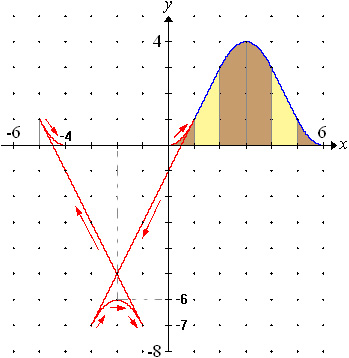图中红色线条部分就是 t3 = -4 的情况。图中的红色箭头表示的是积分方向。因此可见上面的曲线积分中，抛物线（t1）和斜线段（t3）之间由于积分方向不同，存在一些相互抵销作用。从而最终能积到面积 d。这是 t3 的负数解在数学上的含义。但是在物理意义上这样是很难理解的，因为从抛物线片段后，出现了“负时间”，即时间倒流，最终列车停下来时是出发前 4 秒中。因为向着 t 轴负方向，所以 a = dv/dt 并未发生突变。而这种情况在物理意义上比较难以理解。

为了更好理解，换一种方式，把上图中的斜线段部分全部向 t 的正方向映射（由于做了镜面映射，dx = -dx' 所以对应线段上的积分方向同时会被改变），从而上图会演化成下面的图形：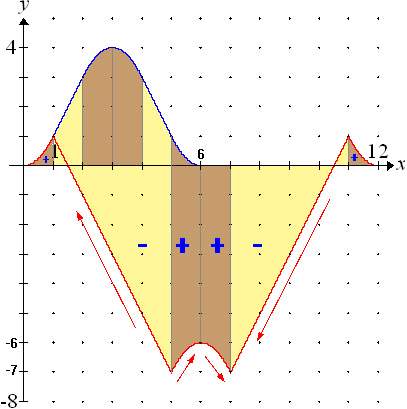在上图中，如果把 t3 视作正数，则意味着加速度在每个衔接点处发生突变（即列车受到无穷大作用力，或者列车质量忽略不计，可看作没有质量没有惯性的质点），显然这也是超现实的。如果不考虑图中的积分方向，则曲线形成的面积显然比 d 大，因此图中标示出了积分方向，实际上两部分是相互抵销作用（斜线部分的积分是 24，抛物线部分的积分是 -12，因此最终两者累加结果是 d = 12）。

尽管 t3 的负数解在物理上没有令人满意的含义，但这就是满足 v 的积分 = d 的数学上的另一种可能情况。

# （二）ZOJ 1086： Octal Factions

题意：给出一个八进制的小数，转换为 10 进制小数。输出格式是：0.d1d2d3 ... dk  = 0.D1D2D3 ... Dm ；

例如对八进制小数0.75，转换为10进制，输出格式：0.75  = 0.953125 。

分析：整数的进制转换的方法非常简单，而这道题目是小数进制转换，把 8 进制转换到 10 进制的直接方法是：

Decimal = d1 * 8^(-1) + d2 * 8^(-2) + ... + dk * 8 ^(-k)

例如对 0.75 （8进制），十进制小数 = 7 / 8  + 5 / 64 = 0.963125;

但是仅仅从题目给出的样例输出来看，语言本身的内置数据类型 double 的精度可能已经不能满足要求了，因此这道题目看起来还是类似大数运算一类的题目，需要借助一个数组来存储数位。但是如果用上面的直接转换方法从 8 进制转换到 10 进制，用数组的方法，在编码实现上是很不方便的。所以考虑以 2 进制小数作为中介，即先把 8 进制转换到 2 进制小数，再转换到 10 进制。后面我们就能看到，这样我们就能很方便的利用数组来保存和给出具有任意精度（取决于数组大小，当然，如果追求更高精度也会引入少许时间和空间的代价）的结果。

把 8 进制小数转换到 2 进制小数的方法非常简单，只需要把8进制小数的每一位用三位二进制数表示即可，例如 0.75  = 0.111 101 ; 设 d1 = (b1 b2 b3) ,  d2 = (b4 b5 b6) ：

则对应的：0.d1 d2 d3 ... dk  = 0.b1 b2 b3  b4 b5 b6  ...  b(3k) b(3k+1) b(3k+2) 

这是显然的，因为：d1 = b1 * 4 + b2 * 2 + b3，...

所以 d1 * 8^(-1) + d2 * 8^(-2) + ... + dk * 8 ^(-k) = (b1*4 + b2*2 + b3) / 8 + ... = b1/2 + b2/4 + b3/8 + ... = 0.b1b2b3b4...;

再把这个二进制小数转换到 10 进制。为了精度需要，这里我引入一个数组：

unsigned char Dec[K];  // K 是一个常数，表征精度

这个数组的意义是，如果10进制小数是 0.D1D2D3 ... Dm ，则 Dec[i] = D(i+1); 最终的10进制小数将是这样：

0. Dec  Dec  Dec  ...

打印时只需要在前面加上“0.”前缀，然后从 0 索引开始依次输出其元素即可。

这里必须注意到二进制小数的小数点后第 k 位的基数 (0.5 ^ k) 的一个很重要的特征，即 (0.5 ^ k) 的小数点后的位数个数是 k（之后将全部是 0）。例如：

0.5，0.25，0.125，0.0625，...

注意这个序列，b[ i + 1 ] = b[ i ] / 2; 由于末尾数永远是5，因此每一次除以 2 都会导致递增一位。因此引入另一个辅助存储空间，用于存储 2 进制小数的从小数点后第 1 位到第 K 位的基数（K 取决于需要的精度），显然这是一个矩阵，例如如果取 K = 6，则定义矩阵 A ：

unsigned char A;

经过初始化计算后，A 的内容如下：

5, 0, 0, 0, 0, 0, 0, 0,    // A: 0.5

2, 5, 0, 0, 0, 0, 0, 0,    // A: 0.25

1, 2, 5, 0, 0, 0, 0, 0,    // A: 0.125

0, 6, 2, 5, 0, 0, 0, 0,    // A: 0.0625

0, 3, 1, 2, 5, 0, 0, 0,    // A: 0.03125

0, 1, 5, 6, 2, 5, 0, 0,    // A: 0.015625

它的右上角元素全是0，应该算是一个下三角矩阵。其意义是 A[k] 是二进制小数第 k+1 位的基数，即 A[k] = 1/2^(k+1); 小数位长度是 Length (A[k]) = k+1;

这个矩阵类似做菜时事先准备的材料，在求解之前先计算好备用。

求解过程如下，首先把结果 Dec 数组全部清零，然后对 8 进制的小数的每一位小数，当作三位二进制数，如果对应的位为 1，就把该位的基数（ A[k] ）逐位累加到结果数组 Dec。最后我们在从后（远离小数点的那一侧）向前处理进位即可，这个过程称为规整，即大数算法中最后的步骤。

代码如下：ZOJ_1086_cpp
#include <stdio.h>#include <string.h>/* 计算结果 0.N N ... */unsigned char Dec;unsigned char A; /* A[k] = 1/( 2^(k+1) ) *//* A[k] 's digit length = (k + 1) */void Init(){int i, j, carry = 0;    A = 5;for(i = 1; i < 48; i++)    {for(j = 0; j < (i+1); j++)        {            A[i][j] = (A[i-1][j] + carry) / 2;            carry = (A[i-1][j] & 1) * 10;        }    }}/* 返回 digit 数位长度*/int Convert(char* pOctNum){/* 位索引 */int testNum[] = { 4, 2, 1 };int index = 0, length = 0;int i, j, carry, digit;char* p = pOctNum + 2; /* Ignore "0." */    memset(Dec, 0, sizeof(Dec));while(*p)    {        digit = *p - '0';for(j = 0; j < 3; j++)        {if(digit & testNum[j])            {                length = index*3 + j + 1;for(i = 0; i < length; i++)                    Dec[i] += A[index*3 + j][i];            }        }        ++p;        ++index;    }/* 归整数位 */for(i = length; i > 0; --i)    {if(Dec[i] > 9)        {            carry = Dec[i]/10;            Dec[i-1] += carry;             Dec[i] = Dec[i] - carry * 10;        }    }return length;}int main(int argc, char* argv[]){int length, i;char line;    Init();while(gets(line) != NULL && line != 0)    {        length = Convert(line);        printf("%s  = 0.", line);for(i = 0; i < length; i++)        {            printf("%d", Dec[i]);        }        printf(" \n");    }return 0;}

总结：

（1）上面的方法，引入的辅助数据是二进制小数的基数，这是因为二进制小数在数位上只有 0 ，1 两种可能，这样实际上就是提供的结果就是，我们是否累加某一个基数到结果中。考虑如果我们用辅助空间存储的直接就是 8 进制小数的基础，则首先，我们要准备这样的基数的代码实现就比较麻烦（准备过程同样是大数除以一个小整数的大数运算！！）。另一点很不便的就是每个基数的小数的位长度和所在位之间没有直接的函数关系（而2进制小数的基数具有这样一个直接关系），我们还需要用对应的某一位上的数字去乘以这个基数再进行累加（而二进制小数的基数直接累加即可）。当然主要的麻烦在于第一点。第二点本来就位于大数算法中的过程之中，所以倒不显得困难。

（2）思考上面的累加过程然后规整数位，有一个累加进位的过程，但该过程是不可能使进位持续到整数部分的，即，假设一个小数的进制为 k ( k >= 2 )，则如果小数点后每一位都是最大数字（k - 1），则这个小数在无限长循环下等于1。即：

1 = 0.99999... = 0.11111... = 0.77777...;

该小数为数列的求和：

f (n) = (k-1) * (k^-1 + k^-2 + ... k ^ -n) =   (1 - k^-n) ;

n 趋向无穷大时，lim f(n) = 1;

（3）题目中并没有明确指明或者暗示我们最终结果的精度，我们如果想要得到更高精度可以把数组取得更大，同时会增加内存需求。因此 K 的取值需要逐渐调整然后提交去“试验”。最初我取 K 值为 256，空间需求略大。最终代码中取 K = 48，结果就内存就已经降低到了ZOL上 C 语言的典型内存需求（120 KB左右）。

【注】本文插图由 GrafEq 和 Photoshop CS 共同绘制。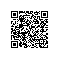使用钉钉扫一扫加入圈子
+ 订阅